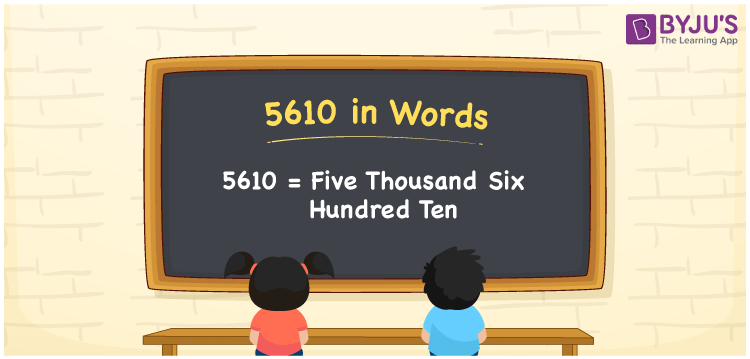# 5610 in Words

5610 in words is written as Five thousand six hundred ten. In both the International System of Numerals and the Indian System of Numerals, 5610 is written as Five thousand six hundred ten. The number 5610 is a Cardinal Number as it could represent some quantity. For example, “this video got 5610 likes”.

 5610 in Words Five thousand six hundred ten Five thousand six hundred ten in Number 5610

## 5610 in English Words

5610 in English words is read as “Five thousand six hundred ten”.## How to Write 5610 in Words?

To write 5610 in words, we shall use the place value chart. In the place value chart, put 5 in the thousands, 6 in the hundreds, 1 in the tens, and 0 in the ones, respectively. Let us make a place value chart to write the number 5610 in words.

 Thousands Hundreds Tens Ones 5 6 1 0

Thus, we can write the expanded form as

5 × Thousand + 6 × Hundred + 1 × Ten + 0 × One

= 5 × 1000 + 6 × 100 + 1 × 10 + 0 × 1

= 5000 + 600 + 10 + 0

= 5610

= Five thousand six hundred ten.

5610 is a natural number, the successor of 5609 and the predecessor of 5611.

5610 in words – Five thousand six hundred ten

• Is 5610 an odd number? – No
• Is 5610 an even number? – Yes
• Is 5610 a perfect square number? – No
• Is 5610 a perfect cube number? – No
• Is 5610 a prime number? – No
• Is 5610 a composite number? – Yes

## Frequently Asked Questions on 5610 in Words

Q1

### How to write 5610 in words?

5610 in words is written as Five thousand six hundred ten.
Q2

### How to write 5610 in the International and Indian System of Numerals?

In both, the system of numerals, 5610 in words, is written as Five thousand six hundred ten.
Q3

### How to write 5610 in a place value chart?

In the place value chart, write 5 in the thousands, 6 in the hundreds, 1 in the tens, and 0 in the ones, respectively.